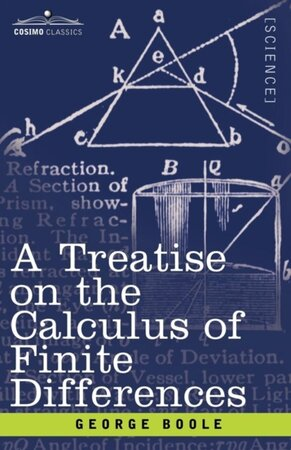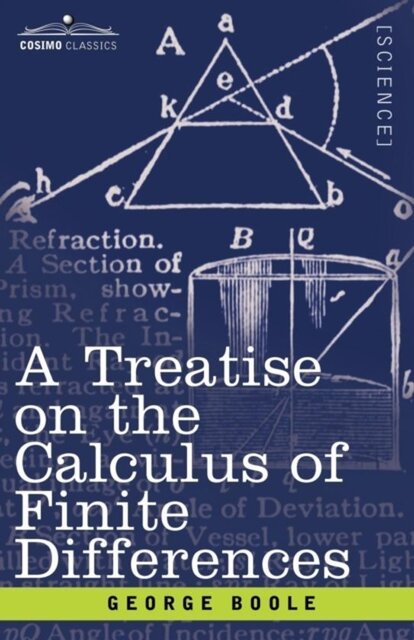Go to top
Voor boeken altijd eerst naar De Slegte

# Boekhandel De Slegte

## Alle vestigingen

Alle vestigingen## Specificaties

• Auteur:
• Uitgever:
Cosimo, Inc.
• ISBN:
9781602063044
• Bindwijze:
Paperback
• Aantal Pagina's:
348
• Genre:
Wetenschap
• Jaar:
2007

# A Treatise on the Calculus of Finite Differences

Beoordeel dit boek als eerste!
Paperback

## Omschrijving

This 1860 classic, written by one of the great mathematicians of the 19th century, was designed as a sequel to his Treatise on Differential Equations (1859). Divided into two sections ("Difference- and Sum-Calculus" and "Difference- and Functional Equations"), and containing more than 200 exercises (complete with answers), Boole discusses: . nature of the calculus of finite differences . direct theorems of finite differences . finite integration, and the summation of series . Bernoulli's number, and factorial coefficients . convergency and divergency of series . difference-equations of the first order . linear difference-equations with constant coefficients . mixed and partial difference-equations . and much more. No serious mathematician's library is complete without A Treatise on the Calculus of Finite Differences. English mathematician and logician GEORGE BOOLE (1814-1864) is best known as the founder of modern symbolic logic, and as the inventor of Boolean algebra, the foundation of the modern field of computer science. His other books include An Investigation of the Laws of Thought (1854).

Volledige beschrijving

## Verkrijgbaar als Tweedehands

Vestiging
Conditie
Prijs
Laatste exemplaar reeds verkocht.

Vestiging